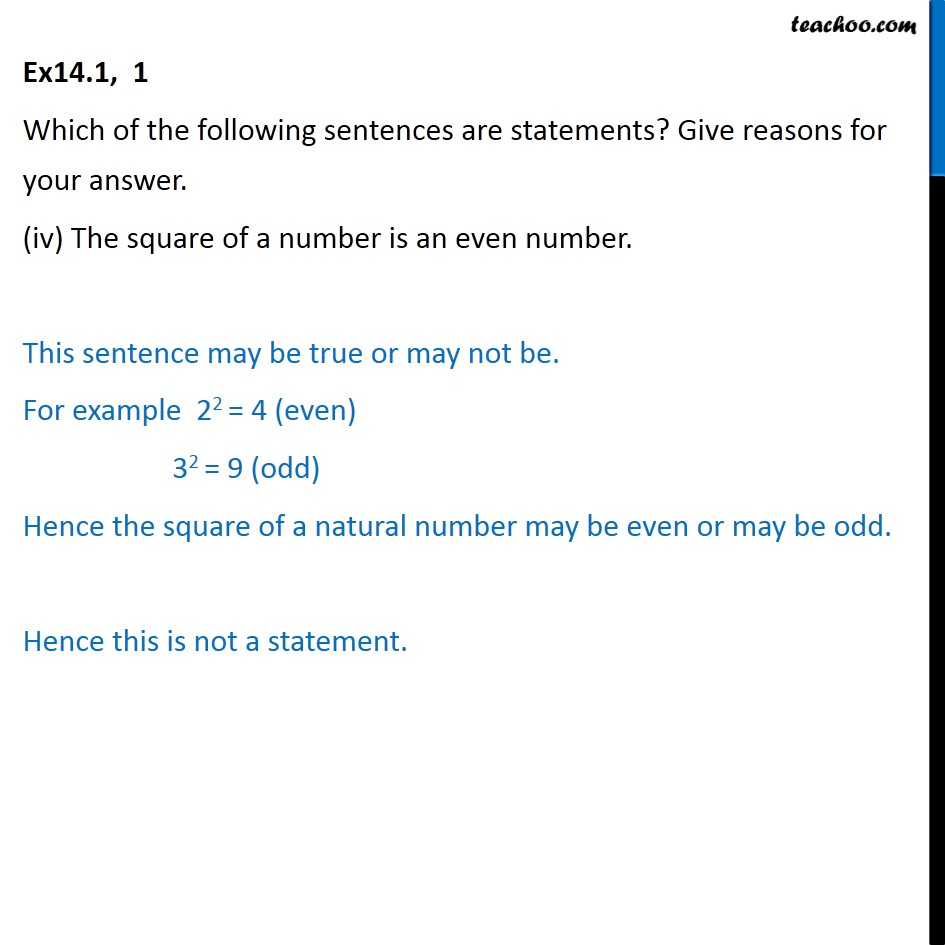1. Chapter 14 Class 11 Mathematical Reasoning (Deleted)
2. Serial order wise
3. Ex 14.1

Transcript

Ex14.1, 1 Which of the following sentences are statements? Give reasons for your answer. (iv) The square of a number is an even number. This sentence may be true or may not be. For example 22 = 4 (even) 32 = 9 (odd) Hence the square of a natural number may be even or may be odd. Hence this is not a statement.